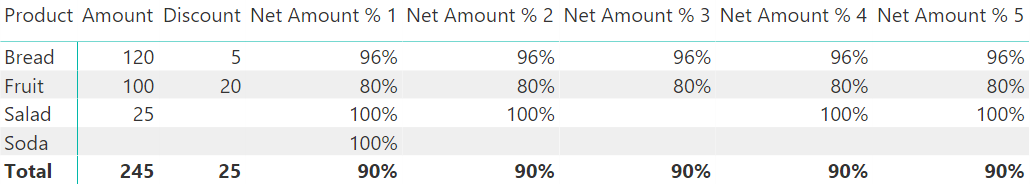## BLANK is not NULL

The first lesson is that BLANK does not correspond to NULL in SQL. The article BLANK Handling in DAX describes this difference with several examples. For this article, it is sufficient to remind the reader that BLANK does not propagate in any operation the way NULL does in SQL. It is important to pay attention to this difference in expressions where an intermediate result could be BLANK.

## Accurate ratios with BLANK measures

Consider a Sales table with Amount and Discount measures providing values for three products (Bread, Fruit, Salad) and no transaction for the Soda product.Without the Products measure, the previous report would have shown only three rows. However, keep in mind that there are four products.

Considering the requirement for an Amount % measure displaying the ratio between the net amount (Amount – Discount) and the Amount value, here are two apparently identical measures that should provide the same result:

```Net Amount % 1 := 1 - ( [Discount] / [Amount] )
Net Amount % 2 := ( [Amount] - [Discount] ) / [Amount]
```

However, for Soda, the resulting report shows a 100% result for the first measure, whereas it shows a blank result for the second measure. The latter is the correct result. Why does this happen? Why is the following equation not valid for DAX?

1 – ( A / B ) = ( B – A ) / B

The reason is that the BLANK value is automatically converted to 0 in sums and subtractions, whereas it propagates as BLANK in divisions and multiplications. The Net Amount % 1 measure first evaluates the ratio between two blank measures for the Soda product. This results in a BLANK,  but in the following subtraction, this BLANK is converted to 0, and the result is 100%. On the contrary, Net Amount % 2  executes the difference between Amount and Discount first. The difference between two blank measures for the Soda product is 0, but the denominator in the following division by Amount is BLANK.This propagates the BLANK to the result of the division.

For these reasons, Net Amount % 2 produces the desired result.

A possible workaround to fix the Net Amount % 1 measure could be the following:

```Net Amount % 2 :=
IF (
NOT ISBLANK ( [Amount] ),
( [Amount] - [Discount] ) / [Amount]
)
```

However, the presence of an IF statement creates a more complex calculation that could potentially be slower. The simpler, the better, also in DAX.

## Accurate ratios with BLANK variables

A best practice is splitting long calculations into smaller steps. This involves using variables to self-document the code and improve performance, avoiding multiple evaluations of the same expressions.

A possible version of the required measure could be the following Net Amount % 3 measure:

```Net Amount % 3 :=
VAR DiscountPercentage =
DIVIDE ( [Discount], [Amount] )
RETURN
IF (
NOT ISBLANK ( DiscountPercentage ),
1 - DiscountPercentage
)
```

However, this version produces a blank result for both Salad and Soda products, whereas we want the same result as for Net Amount % 2. This time, the IF statement testing the DiscountPercentage variable skips Salad because the lack of discounts propagates the BLANK value to that variable, and the following IF statement incorrectly interprets that BLANK as an absence of transactions.The Net Amount % 4 measure provides a good solution based on variables. The advantage of this approach is that every step is well documented. From a performance point of view, the presence of the IF statement involves a cost that could be avoided, but this approach might be required for more complex calculations.

```Net Amount % 4 =
VAR Amount = [Amount]
VAR Discount = [Discount]
VAR DiscountPercentage = Discount / Amount
RETURN
IF (
NOT ISBLANK ( Amount ),
1 – DiscountPercentage
)
```

In this specific case, the simplest approach using variables is the Net Amount % 5 measure, which resembles Net Amount % 2 but guarantees that every measure (Amount and Discount) is evaluated only once.

```Net Amount % 5 =
VAR Amount = [Amount]
VAR Discount = [Discount]
RETURN (Amount - Discount) / Amount
```

## Conclusions

When writing a DAX expression where one or more parts could result in a BLANK value, it is important to pay attention to the different ways in which BLANK propagates. The automatic conversion of BLANK into 0 in sums and subtractions might generate unexpected results. The IF statement adds additional protection, but if omitted for performance reasons,   the calculation must behave as expected – even in all the side cases where a BLANK could appear.

BLANKReturns a blank.

`BLANK ( )`

IFChecks whether a condition is met, and returns one value if TRUE, and another value if FALSE.

`IF ( <LogicalTest>, <ResultIfTrue> [, <ResultIfFalse>] )`